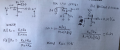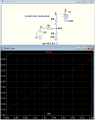# how can i change 0-3.6V to 1.5 to 2.4V?`

#### ashokraj

Joined Feb 1, 2018
133
Hi,
I am looking for a way to change 0-3.6V to 1.5V to 2.4V. are there any possible options? I only have a +ve rail of 2.4V on my board and no negative rail?

#### Ian0

Joined Aug 7, 2020
8,390
IS this an analogue signal? Or a digital signal where 1.5V and 2.4V are the logic levels? What is the frequency?

•drjohsmith

#### ashokraj

Joined Feb 1, 2018
133
IS this an analogue signal? Or a digital signal where 1.5V and 2.4V are the logic levels? What is the frequency?
it is an analogue signal coming from solar panel.

#### ericgibbs

Joined Jan 29, 2010
18,022
hi ash,
Do you have access to any low voltage rail to rail OPA's?
E

#### ashokraj

Joined Feb 1, 2018
133
hi ash,
Do you have access to any low voltage rail to rail OPA's?
E
if needed I can add OPA, but I don't know how to convert it from 0 to 3.6V to range of 1.5 to 2.4

#### Ian0

Joined Aug 7, 2020
8,390
if needed I can add OPA, but I don't know how to convert it from 0 to 3.6V to range of 1.5 to 2.4
Is the solar panel powering the circuit? If so, getting 1.5V out from 0V in might prove a bit challenging.

•BobTPH

#### Alec_t

Joined Sep 17, 2013
13,784
Do you simply want to measure the 0-3.6V and convert the measured value to something in the range 1.5-2.4V; or do you want the converted voltage to power something?

•BobTPH

#### schmitt trigger

Joined Jul 12, 2010
700
Hi,
I am looking for a way to change 0-3.6V to 1.5V to 2.4V. are there any possible options? I only have a +ve rail of 2.4V on my board and no negative rail?
This can be resolved by inspection and superposition, applying resistor equations as shown.
As you have 3 variables and only 2 equations, a common method is to assign a value to one of the variables, let’s say make R2 = 10k, and solve for the rest
I will allow you the pleasure to algebraically solve the equations yourself.Last edited by a moderator:
•ashokraj

#### crutschow

Joined Mar 14, 2008
32,903
Here's an easy way to solve for the resistor values that avoids simultaneous equations calculations.

Solve for the value of R1 when Vin = 3.6V that gives Vout = 2.4V
Since Vout equals the supply voltage, R3 can be ignored, as there is no current through it.

Using the parallel value of R5 with the calculated value for R1, calculate the value for R3 that gives Vout =1.5V for Vin = 0V

Sim below for the calculated values (left as an exercise for the reader).Last edited:
•schmitt trigger and ashokraj

#### MrAl

Joined Jun 17, 2014
10,599
Here's an easy way to solve for the resistor values that avoids simultaneous equations calculations.

Solve for the value of R1 when Vin = 3.6V that gives Vout = 2.4V
Since Vout equals the supply voltage, R3 can be ignored, as there is no current through it.

Using the parallel value of R5 with the calculated value for R1, calculate the value for R3 that gives Vout =1.5V for Vin = 0V

Sim below for the calculated values (left as an exercise for the reader).

View attachment 294600

Hi,

Didnt we have this problem already? Maybe on a different website.

There is something unique about this exact particular problem too and that is that the following relationship holds true (using labels in that drawing):
R5=R1*R3

I think that is unique. That makes me wonder where this problem came from originally (input and output voltage ranges).

#### schmitt trigger

Joined Jul 12, 2010
700
Maybe it is a test question in an undergraduate electrical course?

#### crutschow

Joined Mar 14, 2008
32,903
There is something unique about this exact particular problem too and that is that the following relationship holds true (using labels in that drawing):
R5=R1*R3

I think that is unique.
So you think it's more than just a coincidence?

#### MrAl

Joined Jun 17, 2014
10,599
Maybe it is a test question in an undergraduate electrical course?
Well i have that suspicion and that would make it easy for an instructor to figure out if the student got the right answer.

#### MrAl

Joined Jun 17, 2014
10,599
So you think it's more than just a coincidence?
Hi,

Yes, that's what I was thinking, and it would make it very easy for an instructor to figure out if the student got it right or not, almost by a quick glance.
I can't prove that of course, unless the student comes forward with that information.

It also makes me think there could be a completely different approach to the solution. In that sense it could be just a coincidence, but then again the voltages seem so random, yet they can't be too random.
Maybe we will find out in the future.